# Create A Program to Solve-Table-Based-System in Matlab Assignment Solution.

## Instructions

Objective
Write a program to solve-table-based-system in matlab language.

## Requirements and Specifications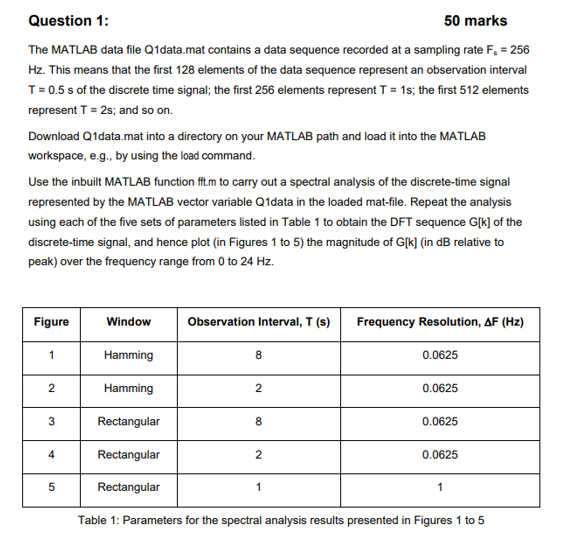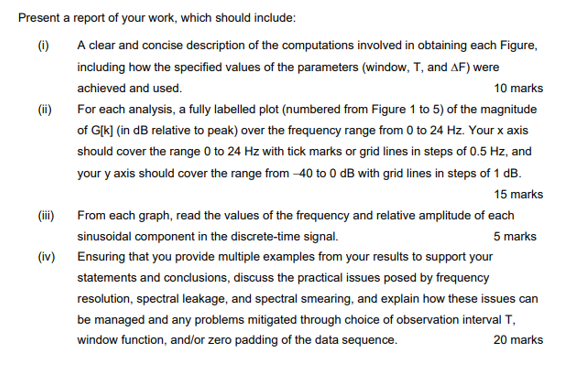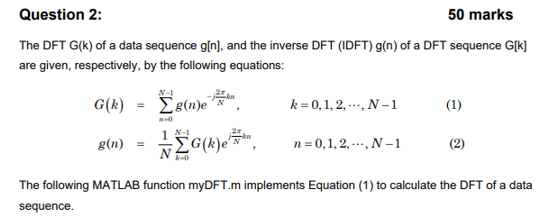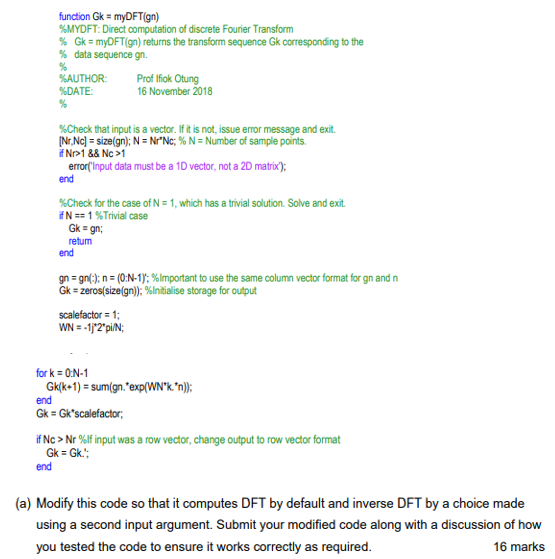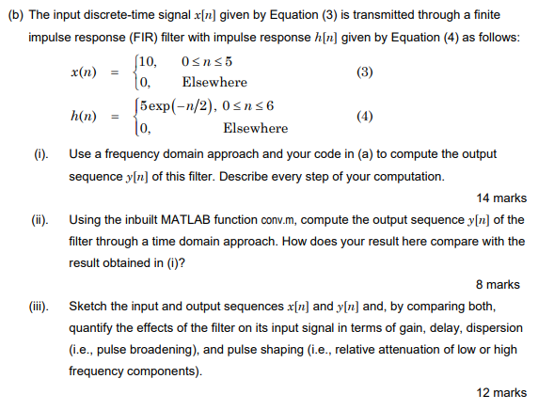Source Code

MY DFT

function Yret = myDFT(Y, varargin)     % First, check if the number of input arguments is 1 or 2     % If the number of input arguments is 1, we assume that the     % input vector is gn and we have to compute Gk. If the number of input arguments in 2 and     % the value of the second argument is "inverse", then we assume that the     % input vector is Gk and we need to compute gn     flag = 0;     if nargin == 1 || (nargin == 2 && strcmp(varargin{1}, 'normal')) % only 1 argument         gn = Y;     elseif nargin == 2 && strcmp(varargin{1}, 'inverse')         Gk = Y;         flag = 1;     else         error('You must define a "normal" FFT transform or "inverse" FFT transform.');     end     if flag == 0 % Compute FFT         [Nr,Nc] = size(gn);         N = Nr*Nc;         if Nr > 1 && Nc > 1             error('Input data must be a 1D vector, not a 2D matrix');         end         if N == 1             Yret = gn;             return;         end         gn = gn(:);         n = (0:N-1)';         Gk = zeros(size(gn));         scalefactor = 1;         WN = -1j*2*pi/N;         for k = 0:N-1             Gk(k+1) = sum(gn.*exp(WN*k.*n));         end         Gk = Gk*scalefactor;         if Nc > Nr             Gk = Gk.';         end         Yret = Gk;     else % compute IFFT         [Nr,Nc] = size(Gk);         N = Nr*Nc;         if Nr > 1 && Nc > 1             error('Input data must be a 1D vector, not a 2D matrix');         end         if N == 1             Yret = Gk;             return;         end         Gk = Gk(:);         k = (0:N-1)';         gn = zeros(size(Gk));         scalefactor = 1/N;         WN = 1j*2*pi/N;         for n = 0:N-1             gn(n+1) = sum(Gk.*exp(WN.*k*n));         end         gn = gn*scalefactor;         if Nc > Nr             gn = gn.';         end         Yret = gn;     end end 

QUESTION 1

clc, clear all, close all % Load data load Q1data.mat y = unnamed; % Define parameters Fs = 256; % in Hz dt = 1/Fs; %% Figure 1 T = 8; % observation time n = T/dt; % number of points y1 = y(1:n); % Portion of signal win = hamming(n); % hamming Window Y1 = fft(y1.*win); % compute fft % Get peak peak = max(Y1); % Convert to dB relative to peak Y1db = 10*log(Y1/peak); dF = 0.0625; % frequency resolution % Frequency vector f = 0:dF:24; L = length(f); figure(1) plot(f, Y1db(1:L)) grid on xlabel('Frequency'); ylabel('Magnitude (dB)') xticks(0:0.5:24) ylim([-40, 0]) xlim([0 24]) %% Figure 2 T = 2; % observation time n = T/dt; % number of points y2 = y(1:n); % Portion of signal win = hamming(n); % hamming Window Y2 = fft(y2.*win); % compute fft % Get peak peak = max(Y2); % Convert to dB relative to peak Y2db = 10*log(Y2/peak); dF = 0.0625; % frequency resolution % Frequency vector f = 0:dF:24; L = length(f); figure(2) plot(f, Y2db(1:L)) grid on xlabel('Frequency'); ylabel('Magnitude (dB)') xticks(0:0.5:24) xlim([0 24]) ylim([-40, 0]) %% Figure 3 T = 8; % observation time n = T/dt; % number of points y3 = y(1:n); % Portion of signal win = rectwin(n); % rect Window Y3 = fft(y3.*win); % compute fft % Get peak peak = max(Y3); % Convert to dB relative to peak Y3db = 10*log(Y3/peak); dF = 0.0625; % frequency resolution % Frequency vector f = 0:dF:24; L = length(f); figure(3) plot(f, Y3db(1:L)) grid on xlabel('Frequency'); ylabel('Magnitude (dB)') xticks(0:0.5:24) ylim([-40, 0]) xlim([0 24]) %% Figure 4 T = 2; % observation time n = T/dt; % number of points y4 = y(1:n); % Portion of signal win = rectwin(n); % rect Window Y4 = fft(y4.*win); % compute fft % Get peak peak = max(Y4); % Convert to dB relative to peak Y4db = 10*log(Y4/peak); dF = 0.0625; % frequency resolution % Frequency vector f = 0:dF:24; L = length(f); figure(4) plot(f, Y4db(1:L)) grid on xlabel('Frequency'); ylabel('Magnitude (dB)') xticks(0:0.5:24) ylim([-40, 0]) xlim([0 24]) %% Figure 5 T = 1; % observation time n = T/dt; % number of points y5 = y(1:n); % Portion of signal win = rectwin(n); % rect Window Y5 = fft(y5.*win); % compute fft % Get peak peak = max(Y5); % Convert to dB relative to peak Y5db = 10*log(Y5/peak); dF = 1; % frequency resolution % Frequency vector f = 0:dF:24; L = length(f); figure(5) plot(f, Y5db(1:L)) grid on xlabel('Frequency'); ylabel('Magnitude (dB)') xticks(0:0.5:24) ylim([-40, 0]) xlim([0 24]) 

QUESTION 2

clc, clear all, close all %% Part a) Test the edited myDFT function     % First, generate a signal with frequencies of 30hz and 70hz % Define sampling frequency Fs = 1000; T = 1/Fs; % period L = 200; % length of signal t = (0:L-1)*T; % time vector % Generate signal y = 5*sin(2*pi*30*t) + 40*sin(2*pi*70*t); % Compute FFT using myDFT Gk = myDFT(y); % Compute the FFT using MATLAB's FFT Gk_fft = fft(y); % Now, restore original signal y_restored = myDFT(Gk, 'inverse'); % To check that the restored signal is correct, we will also restore the % oiginal signal using MATLAB's ifft y_restored_matlab = ifft(Gk); % Now, plot FFT, original and restored signals f = Fs*(0:L/2)/L; Gk2 = abs(Gk/L); Gk1 = Gk2(1:L/2+1); Gk1(2:end-1) = 2*Gk1(2:end-1); Gk2_fft = abs(Gk_fft/L); Gk1_fft = Gk2_fft(1:L/2+1); Gk1_fft(2:end-1) = 2*Gk1_fft(2:end-1); figure subplot(1,3,1) stem(f, Gk1, 'color', 'k', 'linewidth', 2.5), hold on stem(f, Gk1_fft, 'y--', 'linewidth', 1.5) grid on legend('myDFT', "MATLAB's ifft"); title('Frequency Spectrum'); subplot(1,3,2) plot(t, y), grid on title('Original Signal') subplot(1,3,3) hold on plot(t, y_restored, 'color', 'k', 'linewidth', 2) plot(t, y_restored_matlab, 'y--', 'linewidth', 1.5) legend('myDFT', "MATLAB's ifft"); grid on title('Restored Signal') %% Part b) Signal and filter % Generate a vector n from 0 to 10 n = linspace(0, 10, 1000); % Generate signal x = 10*ones(1, length(n)); % For values of n > 5, the value of the signal is zero x(n>5) = 0; % Now, generate h h = 5*exp(-n/2); % For n > 6, set the value of h = 0 h(n>6) = 0; %% Part (i) % Using frequency domain, let's compute the FFT of each signal X = myDFT(x); H = myDFT(h); % Compute output signal. The convolution of two signals in time domain is % equal to the point-wise multiplication of both signals in frequency % domain Y = X.*H; % Now, compute signal in time-domain by calculating the inverse FFT y1 = myDFT(Y,'inverse'); %% Part (ii) % Compute the output using MATLAB's conv y2 = ifft(fft(x).*fft(h)); %% Part (iii) % Now, plot both signals to compare them figure plot(n,y1, 'color', 'k', 'linewidth', 2) hold on plot(n,y2, 'y--', 'linewidth', 1.5) legend('My Output', 'MALTAB conv'); grid on xlabel('n') ylabel('Magnitude')